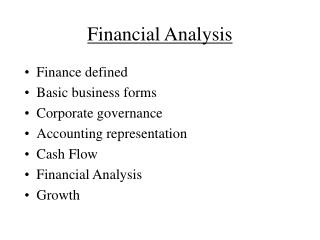# Financial Analysis - PowerPoint PPT PresentationDownload PresentationFinancial Analysis

Financial AnalysisDownload Presentation## Financial Analysis

- - - - - - - - - - - - - - - - - - - - - - - - - - - E N D - - - - - - - - - - - - - - - - - - - - - - - - - - -
##### Presentation Transcript

1. Financial Analysis • Finance defined • Basic business forms • Corporate governance • Accounting representation • Cash Flow • Financial Analysis • Growth

2. Finance • “The science of the motion of money” • Finance and accounting • Finance and economics • Finance and management • Body of knowledge • Basic areas in finance • Investments and financial institutions • Corporate finance • NWC • LT financing • Long term investment

3. Forms of organizations • Sole proprietorship, partnership, corporation • Advantages • Disadvantages • Focus of the course: CORPORATION • Snapshot of a corporation • Right side • Left side

4. Corporate Governance • 4 actors: • Shareholders • Creditors • Officers • Stakeholders • 1 GOAL? • Agency problems = Cost • Direct (compensations and perquisites) • Indirect (monitoring and non-optimal decision making)

5. Financial position • Income Statement (over a period) NI=(Sales-COGS-Dpr-Int) X (1-T) • Retained Earnings(over a period) End RE=Beg. RE +NI - Div • Balance Sheet (At one point in time) • Assets=Liabilities + Equity = Liability + Stocks + RE

6. Cash flow • Define sources and uses of cash • Another (smarter) way to look at cash flow: • Cash flow from assets=CF to creditors +CF to owners • Cash from asset = Operating cash flow = EBIT-T+Dpr - Use of NWC = End NWC-Beg.NWC - Long term asset spending = End NFA - Beg.NFA + Dpr • Cash to creditor = Interest paid –net new borrowing • Cash to owners = dividends paid- net new equity raised • Calculate and interpret (from Hermetic Inc.)

7. Sources and Uses of Cash

8. Another way to look at Cash flows

9. Financial Statements Analysis • ISREBSCF • Common size analysis • Ratio analysis • Cross sectional analysis • Time serie analysis • 5 families • ST Liquidity • LT Liquidity • Activity • Profitability • Market

10. Ratio analysis • ST Liquidity: • Current ratio=CA/CL • Acid test=(CA-Inv.)/CL • LT Liquidity: • Debt-to-equity ratio=D/E • Cash coverage ratio= (EBIT+Dpr)/Int • Activity: • Short-term asset turnover • Asset turnover=Sales/Asset • Profitability • Profit margin=NI/Sales • ROA=NI/Asset • ROE=NI/Equity

11. Dupont Equation • ROE= NI/Sales x Sales/Assets x Assets/Equity • ROE= Profit x Asset x Equity Margin Turnover Multiplier • Operating efficiency • Asset use efficiency • Financial leverage • ROE=ROA x Equity multiplier • Differentiate ROE and ROA • Remedy to “paralysis by analysis”

12. Growth • Internal growth: Debt is constant • g= ROA x b/(1-ROA x b) • Sustainable growth: Debt to equity is constant • g=ROE x b/(1-ROE x b) • Differentiate between internal and sustainable growth. • What are the factors that affect growth? • Forecasting tool

13. Cases Study Sunset Board S&S Air